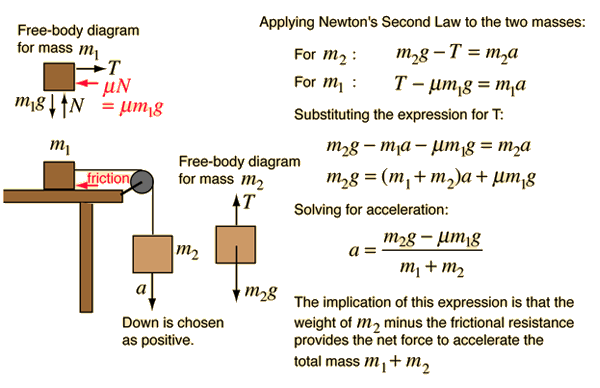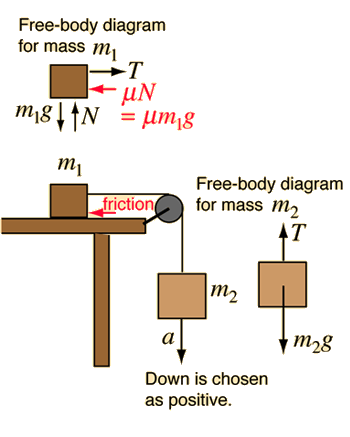# Horizontal Pulley with Friction### Calculation****Remove friction

Index

Newton's laws

Standard mechanics problems

 HyperPhysics***** Mechanics R Nave
Go Back

# Horizontal Pulley with Friction

Application of Newton's second law to a horizontal pulley. Note that the tension in the rope is NOT equal to the weight of the hanging mass except in the special case of zero acceleration.Given a mass of kg on a horizontal table. It is attached by a rope over a pulley to a mass of kg which hangs vertically. The friction between the mass and the surface is represented by a friction coefficient mu= . Taking downward as the positive direction for the hanging mass, the acceleration will be

### Acceleration =m/s²

With this acceleration, the tension in the rope will be
T= Newtons compared to the weight W = Newtons for the hanging mass. If the weight of the hanging mass is less than the frictional resistance force acting on the mass on the table, then the acceleration will be zero.

### Expressions****Remove friction

Index

Newton's laws

Standard mechanics problems

 HyperPhysics***** Mechanics R Nave
Go Back